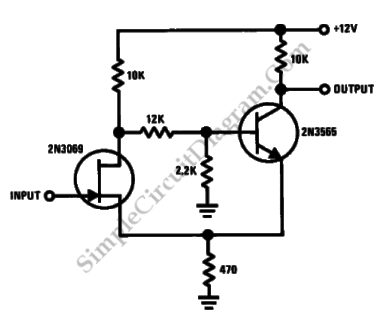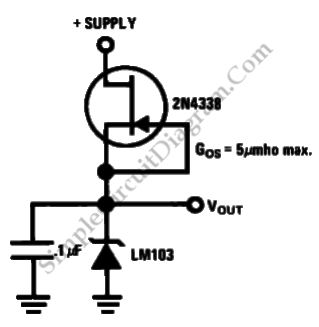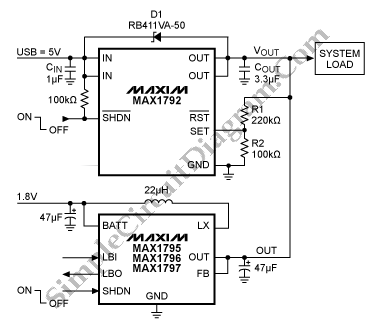## Discrete Schmitt TriggerThis is a schmitt trigger circuit. This circuit produces a simple comparator action. It is “emitter coupled”. This circuit has a distinct hysteresis loop and fast transition action because this circuit uses the 2N3565 bipolar that is a high hFE transistor. The 2N3069 JFET generates very little loading on the measured input. Here is the circuit : [Source: National Semiconductor […]

## Low Power Regulator ReferenceThis is a simple circuit of low power regulator reference. This circuit can produces stable voltage reference. The voltage reference that is produced by this circuit  almost free of supply voltage hash. This circuit has typical power supply rejection go over 100dB. Here is the circuit : [Source: National Semiconductor Application Note]

## Precision Current SourceThis is a circuit of precision current source. This circuit uses two kinds of transistor, the 2N2219 bipolar and the 2N3069 JFET. Both of them are used as voltage devices between the current sensing resistor and the output. As the current sensing resistor this circuit uses R1. Here is the circuit : This circuit is called current source because this […]